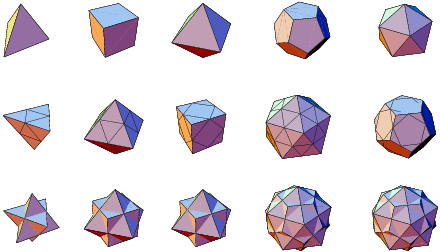# How does it relate?

Probability Level 2What is the coefficient of $x^{99}$ in the expansion of $(x+1)(x+2)(x+3)\cdots (x+100)?$

The challenge is to relate the problem with combinatorics and the given picture and to provide a solution that is based on combinatorics rather than algebra.

×